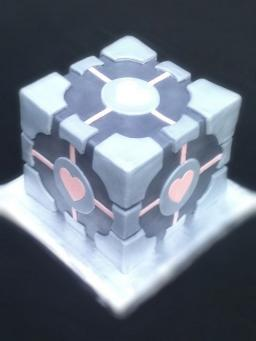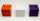# Volume from surface area

What is the volume of the cube whose surface area is 96 cm2?

V =  64 cm3

### Step-by-step explanation:Did you find an error or inaccuracy? Feel free to write us. Thank you!Tips to related online calculators
Tip: Our volume units converter will help you with the conversion of volume units.

## Related math problems and questions:

• BallsThree metal balls with volumes V1=71 cm3 V2=78 cm3 and V3=64 cm3 melted into one ball. Determine it's surface area.
• Cube 6Volume of the cube is 216 cm3, calculate its surface area.
• Prism XThe prism with the edges of the lengths x cm, 2x cm, and 3x cm has volume 20250 cm3. What is the area of the surface of the prism?
• Equilateral cylinderEquilateral cylinder (height = base diameter; h = 2r) has a volume of V = 199 cm3 . Calculate the surface area of the cylinder.
• Cuboids in cubeHow many cuboids with dimensions of 6 cm, 8 cm and 12 cm can fit into a cube with side 96 centimeters?
• Three cubesThe body was created by gluing three identical cubes. Its volume is 192 cm3. What is its surface in dm2?
• Cube 1-2-3Calculate the volume and surface area of the cube ABCDEFGH if: a) /AB/ = 4 cm b) perimeter of wall ABCD is 22 cm c) the sum of the lengths of all edges of the cube is 30 cm.
• Cube volumeThe cube has a surface of 384 cm2. Calculate its volume.
• Cube wallsFind the volume and surface area of the cube if the area of one wall is 40cm2.
• Cube diagonalsDetermine the volume and surface area of the cube if you know the length of the body diagonal u = 216 cm.
• Center of the cubeThe Center of the cube has a distance 16 cm from each vertex. Calculate the volume V and surface area S of the cube.
• Cube surface and volumeThe surface of the cube is 500 cm2, how much cm3 will be its volume?
• Surface and volume - cubeFind the surface and volume of a cube whose wall diagonal is 5 cm long.
• Cube surface areaThe Wall of the cube has a content area 99 cm square. What is the surface of the cube?
• Magnified cubeIf the lengths of the cube's edges are extended by 5 cm, its volume will increase by 485 cm3. Determine the surface of both the original and the magnified cube.
• Cuboid wallsCalculate the cuboid volume if its different walls have an area of 195cm², 135cm², and 117cm².
• Three cubesTwo cube-shaped boxes with edges a = 70 cm; b = 90 cm must be replaced by one cube-shaped box. What will be its edge?